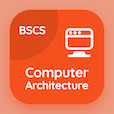Class 10 Online Courses

Grade 10 Physics MCQ PDF - Chapters

# Geometrical Optics MCQ Questions Online p. 6

Practice Geometrical optics MCQ with answers, geometrical optics quiz questions with answers PDF to solve grade 10 physics MCQ bank 6 for online classes. Lenses and Characteristics Multiple Choice Questions and Answers (MCQs), geometrical optics quiz questions and answers for online school and college. "Geometrical Optics MCQ" PDF eBook: lenses and characteristics, telescope, spherical mirrors, image formation by lenses test prep for online school and college.

"Concave lens is also called" Multiple Choice Questions (MCQ) on geometrical optics with choices converging lens, diverging lens, convex lens, and none of above for online school and college. Solve lenses and characteristics quiz questions for school certificate programs for online school courses.

## MCQs on Geometrical Optics

MCQ: Concave lens is also called

diverging lens
converging lens
convex lens
none of above

MCQ: To see dim stars, we use

simple microscope
compound microscope
endoscope
telescope

MCQ: Power of a lens is defined as the reciprocal of its

focal length in meters
principal axis
optical center
vertex

MCQ: The focus lies behind the mirror in

convex mirror
concave mirror
silver mirror
plane mirror

MCQ: After refraction by the lens, the ray parallel to the principal axis passes through the

optical center
vertex
focal point
core